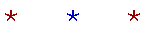Proyecto Salón Hogar

English SectionMath Page

# CALCULUS

Home

The Lessons

12.  Limits

A sequence of rational numbers. The limit of a variable. The continuity of the x-axis.
The limit of a function at a point. Theorems on limits. Limits of polynomials.

The definition of "continuous at a point."

The definition of "becomes infinite." Limits of rational functions.

The slope of a tangent line to a curve. The difference quotient and the definition of the derivative. Differentiability. Notations for the derivative.

The derivative of a constant. The derivative of y = x. The product rule.
The power rule.
The derivative of the square root.

The derivative of a function of a function.

The quotient rule.
Implicit differentiation.
The derivative of inverse functions.

Instantaneous velocity.
Related rates.

The turning points of a graph. Critical values.

Exponential growth. The system of natural logarithms. The general power rule.

15.  Evaluating e

 Appendix. What is a number? The existence of the real numbers.Home

www.proyectosalonhogar.com